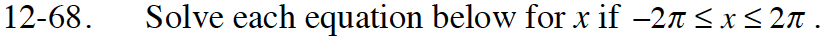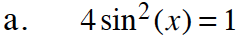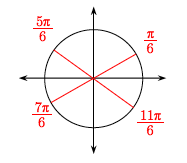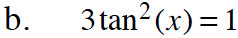Home > CCA2 > Chapter 12 > Lesson 12.1.4 > Problem12-68

12-68.
1. Solve each equation below for x if −2πx ≤ 2π. Homework Help ✎

1. 4 sin2(x) = 1

2. 3 tan2(x) = 1$\sin^2(x)\ =\frac{1}{4}$

Divide both sides by 4.

Take the square root of both sides to solve for sin(x).

$\sin(x)\ =±\frac{1}{2}$

Find the corresponding angles on the unit circle.Follow the steps in part (a).